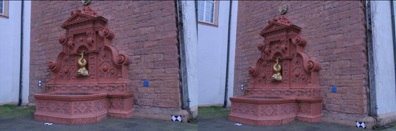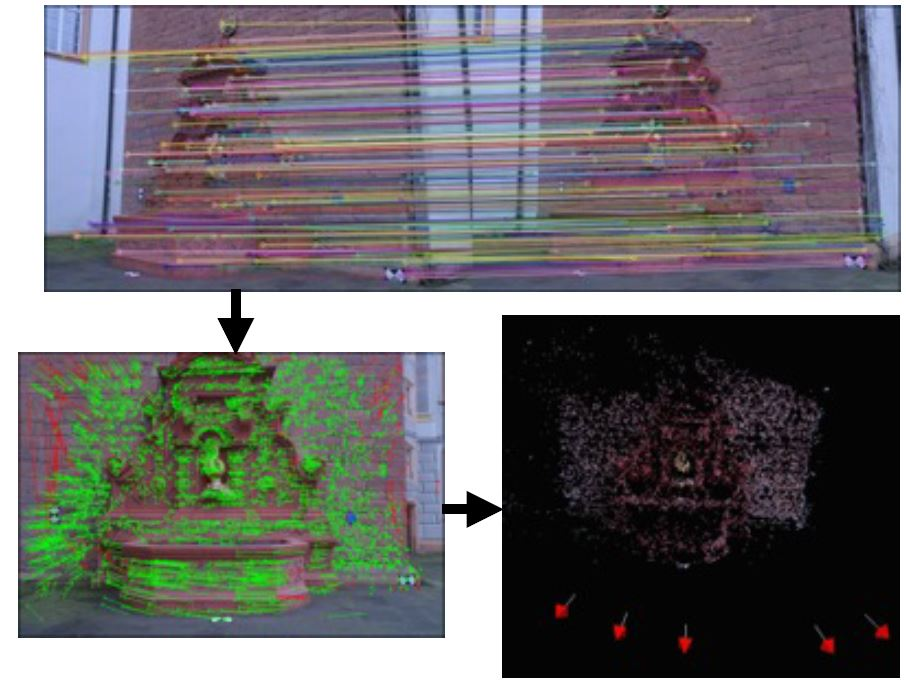Posted on

# Structure from MotionJan Handzus

Main objective of this project was to reconstruct the 3D scene from set of images or recordedÂ video. First step is to find relevant matches between two related images and use this matches toÂ calculate rotation and translation of camera for each input image or frame. In final stage the depthÂ value is extracted with triangulation algorithm.

## INPUT## THE PROCESS

1. Find features in two related images:
```SurfFeatureDetector detector(400);
detector.detect(actImg, keypoints1);
detector.detect(prevImg, keypoints2);
```
2. Create descriptors for features:
```SurfDescriptorExtractor extractor(48, 18, true);
extractor.compute(actImg, keypoints1, descriptors1);
extractor.compute(prevImg, keypoints2, descriptors2);
```
3. Pair descriptors between two images and find relevant matches:
```BFMatcher matcher(NORM_L2);
matcher.match(descriptors1, descriptors2, featMatches);
```
4. After we have removed the irrelevant key-points we need to extract the fundamental matrix:
```vector<Point2f> pts1,pts2;
keyPointsToPoints(keypoints1, pts1);
keyPointsToPoints(keypoints2, pts2);
Fundamental = findFundamentalMat(pts1, pts2, FM_RANSAC, 0.5, 0.99, status);
```
5. Calculate the essential matrix:
```Essential = (K.t() * Fundamental * K);
```

Kâ€¦. the camera calibration matrix.

6. First camera matrix is on starting position therefore we must calculate second camera matrix P1:
```SVD svd(Essential, SVD::MODIFY_A);
Mat svd_u = svd.u;
Mat svd_vt = svd.vt;
Mat svd_w = svd.w;
Matx33d W(0, -1, 0,
1, 0, 0,
0, 0, 1);
//Rotation
Mat_<double> R = svd_u * Mat(W) * svd_vt;
//Translation
Mat_<double> t = svd_u.col(2);
```
7. Find depth value for each matching point:
```//Make A matrix.
Matx43d A(u.x*P(2, 0) - P(0, 0), u.x*P(2, 1) - P(0, 1), u.x*P(2, 2) - P(0, 2),
u.y*P(2, 0) - P(1, 0), u.y*P(2, 1) - P(1, 1), u.y*P(2, 2) - P(1, 2),
u1.x*P1(2, 0) - P1(0, 0), u1.x*P1(2, 1) - P1(0, 1), u1.x*P1(2, 2) - P1(0, 2),
u1.y*P1(2, 0) - P1(1, 0), u1.y*P1(2, 1) - P1(1, 1), u1.y*P1(2, 2) - P1(1, 2)
);
//Make B vector.
Matx41d B(-(u.x*P(2, 3) - P(0, 3)),
-(u.y*P(2, 3) - P(1, 3)),
-(u1.x*P1(2, 3) - P1(0, 3)),
-(u1.y*P1(2, 3) - P1(1, 3)));
//Solve X.
Mat_<double> X;
solve(A, B, X, DECOMP_SVD);
return X;
SVD svd(Essential, SVD::MODIFY_A);
Mat svd_u = svd.u;
Mat svd_vt = svd.vt;
Mat svd_w = svd.w;
Matx33d W(0, -1, 0,
1, 0, 0,
0, 0, 1);
//Rotation
Mat_<double> R = svd_u * Mat(W) * svd_vt;
//Translation
Mat_<double> t = svd_u.col(2
```

## SAMPLE## CONCLUSION

We have successfully extracted the depth value for each relevant matching point. But we were not able to visualise the result because of the PCL and other external libraries. In future we try to use Matlab to validate our result.